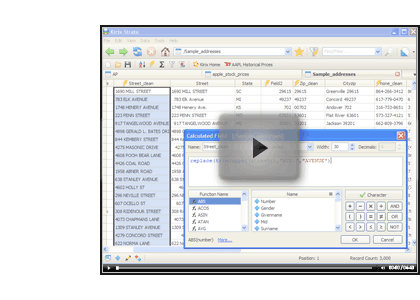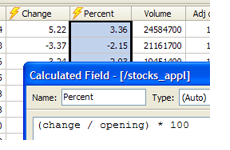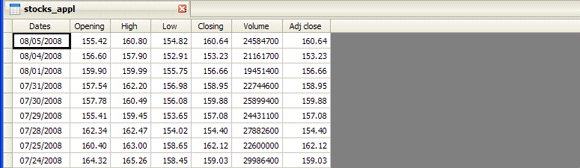Calculated Fields | Kirix Strata Blog

# Kirix Strata Blog

## Archive for the ‘calculated fields' Category

### JD Edwards Date Conversions (CYYDDD)

Thursday, April 16th, 2009

In the last post, we talked about Julian dates and Unix Timestamp conversion. We recently received a support request regarding another type of date conversion and, since it might be applicable to others, we thought it would be a good idea to show how you can convert it in Strata. The format is C-YY-DDD, which is used by JD Edwards software: the century after 1900, the year in that century and the Julian date within that year. Here are a few examples:

```105031 - January 31, 2005
107263 - September 20, 2007
98129 - May 9, 1998```

To convert the dates we first want to get the string into the proper year and then we'll add the Julian date to get the proper day/month (which will also take into account leap years). So, let's take the example “107263″ (we'll assume that it is in Numeric format in your field). Here is the full expression you can use in a calculated field:

`date(1900+(field1/1000),1,1)+val(right(str(field1),3))-1`

### Getting the Year

`date(1900+(field/1000),1,1)`

The first part of the formula takes the string and divides by 1000, which, when rounded, will provide the first three digits (”107). It then adds 1900 to the 107 to give us “2007″. Then the normal date conversion applies and we end up with “01/01/2007.”

### Getting the Day and Month

`val(right(str(field1),3))-1`

Here we are adding the Julian days for the year to the date we created above “01/01/2007″ — so, if we added “1″ to this date, we would get 01/02/2007, and so on. Here we are using the RIGHT() function to pull out the 3 digits on the right side of the field, in this case “263.” Because it was numeric, we needed to first convert it to a string using the STR() function and then we converted the result back to a numeric value using the VAL() function. This gives us “09/21/2007.” Because we started at “1″ (January 1st) instead of “0″, we simply need to subtract 1 from our date to obtain the correct date of “09/20/2007.”

NOTE: If your JDE date was a character field instead of a numeric field to begin with, you could change the structure or just convert manually in your expression with the VAL() function as follows:

`date(1900+(val(field3)/1000),1,1)+val(right(str(val(field3)),3))-1`

If anyone has other date conversions they're having trouble with, please let us know and we'll see if we can help.

### Tutorial: Creating Calculations and Cleaning up Dirty Data

Tuesday, December 16th, 2008

Continuing with our video tutorial series, here's one on creating calculations and using formulas. This one briefly looks at how to create calculated fields, but quickly delves into more complex formulas and functions. This one would be worth watching, even if you're an expert — it may give you a few new ideas.### Exploring Calculated Fields: Part 2 - Extracting Data Values with STRPART()

Thursday, August 14th, 2008

In part 1 of this series, we looked at the basics of using calculated fields, including referencing fields and using functions.

In this installment, we'll look at how you can use calculated fields to extract data values from a column with “dirty” data. This happens more than one might think: if you've ever "cleaned" a list of contact information or migrated databases, you know that you often have to take a column and break it up into multiple parts. Calculated fields make this task very easy.

Let's look at an example. Suppose we have a table that contains raw contact information smashed together in a single field:Let's extract each of these parts — first name, last name and email address — from the single column into multiple columns. We'll do this by using a calculated field with a function that parses the string, based on a delimiter (such as a space), and returns the relevant portion of the string. (more…)

### Exploring Calculated Fields: Part I - The Basics

Wednesday, August 6th, 2008Calculated fields are one of the cornerstones of data analysis. It's just one of those tools that you end up using again and again. And, if you can master the functions and formulas and make them do your bidding, you'll be well on your way to becoming a data Jedi. This article begins a multi-part blog series on using calculated fields in your projects.

Calculated fields are nothing more than a way to apply a formula to existing columns or fields. (As a quick aside, spreadsheets use the terms row/column, whereas databases use the terms record/field — there are some technical differences, but for our purposes here, they are very similar). A poor man's calculated field is found in a spreadsheet; you create a formula in a blank cell and then drag the cell down to apply it to the other rows. The more traditional calculated field is found in the database world. Here you create a new field that encapsulates a calculation and then you run it across the entire table.

With Kirix Strata, you get a calculated field like that found in the database world, but with the dynamic/instant properties you get in the spreadsheet world. So, when you create a calculated field in Strata, you get a calculation that applies to the entire data set instantly — whether its 10 rows or 10 million. In addition, these fields behave like regular ol' fields, so you can reference them in other calculated fields or use them in your sorts, filters, groups, relationships, queries and other operations. Of course, the actual data can't be edited like in a regular field, but the underlying formula can be tweaked — with the results showing up in your view in real-time.

Let's create some simple examples to show how calculated fields work. We'll look at the historic stock prices of Apple Computer (to try this yourself, just click the “Download to Spreadsheet” at the bottom of the web page and it will open up into a table in Strata):